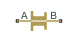# Fixed Orifice with Fluid Inertia

(To be removed) Fixed hydraulic orifice accounting for flow inertia

The Hydraulics (Isothermal) library will be removed in a future release. Use the Isothermal Liquid library instead. (since R2020a)

## Library

Orifices

•## Description

The Fixed Orifice with Fluid Inertia block models a hydraulic fixed orifice and accounts for the fluid inertia, in addition to the static pressure loss.

Fluid inertia plays a noticeable role in orifices with a large ratio of orifice length to the orifice hydraulic diameter (L / DH) and in sharp-edged short orifices when the rate of change of flow rate (fluid acceleration) is relatively large.

The orifice is based on the following equations:

`$q={C}_{D}\cdot A\sqrt{\frac{2}{\rho }}\cdot \frac{{p}_{r}}{{\left({p}_{r}^{2}+{p}_{cr}^{2}\right)}^{1/4}}$`
`$p={p}_{in}+{p}_{r}$`
`${p}_{in}=\rho \frac{L}{A}\frac{dq}{dt}$`

where

 q Volumetric flow rate p Total pressure differential pin Inertial pressure drop pr Resistive pressure drop CD Flow discharge coefficient A Orifice passage area L Orifice length ρ Fluid density pcr Minimum pressure for turbulent flow

The minimum pressure for turbulent flow, pcr, is calculated according to the laminar transition specification method:

• By pressure ratio — The transition from laminar to turbulent regime is defined by the following equations:

pcr = (pavg + patm)(1 – Blam)

pavg = (pA + pB)/2

where

 pavg Average pressure between the block terminals patm Atmospheric pressure, 101325 Pa Blam Pressure ratio at the transition between laminar and turbulent regimes (Laminar flow pressure ratio parameter value)
• By Reynolds number — The transition from laminar to turbulent regime is defined by the following equations:

`${p}_{cr}=\frac{\rho }{2}{\left(\frac{{\mathrm{Re}}_{cr}\cdot \nu }{{C}_{D}\cdot {D}_{H}}\right)}^{2}$`
`${D}_{H}=\sqrt{\frac{4A}{\pi }}$`

where

 DH Orifice hydraulic diameter ν Fluid kinematic viscosity Recr Critical Reynolds number (Critical Reynolds number parameter value)

Connections A and B are conserving hydraulic ports associated with the orifice inlet and outlet, respectively. The block positive direction is from port A to port B. This means that the flow rate is positive if it flows from A to B, and the pressure differential is determined as $\Delta p={p}_{\text{A}}-{p}_{\text{B}},$.

## Parameters

Orifice area

Cross-sectional area of the orifice. The default value is `1e-4` m^2.

Orifice length

Total length of the orifice. Generally, increase the geometrical length of the orifice up to 2 · 0.8 · DH (where DH is the orifice hydraulic diameter) to take into account the added volumes of fluid on both sides of the orifice. The default value is `0.01` m.

Flow discharge coefficient

Semi-empirical parameter for orifice capacity characterization. The coefficient affects the resistive pressure drop in the orifice. The default value is `0.6`.

Laminar transition specification

Select how the block transitions between the laminar and turbulent regimes:

• `Pressure ratio` — The transition from laminar to turbulent regime is smooth and depends on the value of the Laminar flow pressure ratio parameter. This method provides better simulation robustness.

• `Reynolds number` — The transition from laminar to turbulent regime is assumed to take place when the Reynolds number reaches the value specified by the Critical Reynolds number parameter.

Laminar flow pressure ratio

Pressure ratio at which the flow transitions between laminar and turbulent regimes. The default value is `0.999`. This parameter is visible only if the Laminar transition specification parameter is set to `Pressure ratio`.

Critical Reynolds number

The maximum Reynolds number for laminar flow. The value of the parameter depends on the orifice geometrical profile. You can find recommendations on the parameter value in hydraulics textbooks. The default value is `10`. This parameter is visible only if the Laminar transition specification parameter is set to `Reynolds number`.

Initial flow rate

Flow rate through the orifice at the start of simulation. This parameter specifies the initial condition for use in computing the block's state at the beginning of a simulation run. For more information, see Initial Conditions Computation. The default value is `0`.

## Global Parameters

Parameters determined by the type of working fluid:

• Fluid density

• Fluid kinematic viscosity

Use the Hydraulic Fluid block or the Custom Hydraulic Fluid block to specify the fluid properties.

## Ports

The block has the following ports:

`A`

Hydraulic conserving port associated with the orifice inlet.

`B`

Hydraulic conserving port associated with the orifice outlet.

## Version History

Introduced in R2013a

expand all